# TRUE NCERT SOLUTIONS

TRUE NCERT SOLUTIONS published 29 applications on Google Play, 1,155 people rated these apps with an average rating of 2.44!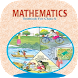10th Maths NCERT Solution

Rated 4.65(258) — TRUE NCERT SOLUTIONS

???? Offline solution book of NCERT class 10th CBSE mathematics textbook.This app provides solution of all chapters and exercise of CBSE class 10th NCERT Maths Textbook. This app is very easy to use. You have to just know the chapter and exercise number..

Free App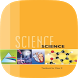10th Science NCERT Textbook

Rated 4.73(11) — TRUE NCERT SOLUTIONS

Offline textbook of class 10th NCERT science .We will be constantly updating this app and adding more features by getting feedback from users. So if you have any suggestion or found any bugs in the app, let us know as soon as possible. We will quickly...

Free App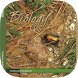11th Biology NCERT Textbook

Rated 0.00(0) — TRUE NCERT SOLUTIONS

NCERT Textbook of class XI Biology (CBSE Board). Works without Internet.

Free App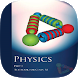11th Physics NCERT Solution

Rated 0.00(0) — TRUE NCERT SOLUTIONS

NCERT Solution of class 11 Physics (CBSE Board). Works without Internet.

Free App12th Maths NCERT Solution

Rated 4.68(91) — TRUE NCERT SOLUTIONS

???? Offline solution book of NCERT class 12th CBSE mathematics textbook.This app provides solution of all chapters and exercise of CBSE class 12th NCERT Maths Textbook. This app is very easy to use. You have to just know the chapter and exercise number..

Free App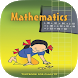6th Maths NCERT Textbook

Rated 0.00(0) — TRUE NCERT SOLUTIONS

NCERT Solution of class 6 Mathematics Textbook (CBSE Board). No Internet.

Free App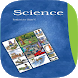6th Science NCERT Textbook

Rated 0.00(0) — TRUE NCERT SOLUTIONS

NCERT Solution of class 6 Science Textbook(CBSE Board). Works without Internet.

Free App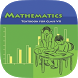7th Maths NCERT Textbook

Rated 0.00(0) — TRUE NCERT SOLUTIONS

NCERT Textbook of class 7 Maths (CBSE Board). Works without Internet.

Free App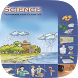7th Science NCERT Textbook

Rated 0.00(0) — TRUE NCERT SOLUTIONS

NCERT Textbook of class 7 Science (CBSE Board). Works without Internet.

Free App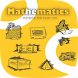8th Maths NCERT Textbook

Rated 4.29(14) — TRUE NCERT SOLUTIONS

NCERT Textbook of class 8 Maths (CBSE Board). Works without Internet.

Free App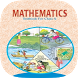10th Maths NCERT Textbook

Rated 0.00(0) — TRUE NCERT SOLUTIONS

NCERT Textbook of class 10 Maths (CBSE Board). Works without Internet.

Free App11th Biology NCERT Solution

Rated 0.00(0) — TRUE NCERT SOLUTIONS

NCERT Solution of class XI Biology Solution CBSE Board). Works without Internet.

Free App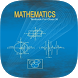11th Maths NCERT Solution

Rated 4.00(30) — TRUE NCERT SOLUTIONS

???? Offline solution book of NCERT class 11th CBSE mathematics textbook.This app provides solution of all chapters and exercise of CBSE class 11th NCERT Maths Textbook. This app is very easy to use. You have to just know the chapter and exercise number..

Free App11th Physics NCERT Textbook

Rated 0.00(0) — TRUE NCERT SOLUTIONS

NCERT textbook for class 11 Physics (CBSE Board). Works without Internet.

Free App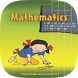6th Maths NCERT Solution

Rated 4.65(204) — TRUE NCERT SOLUTIONS

???? Offline solution book of NCERT class 6th CBSE mathematics textbook.This app provides solution of all chapters and exercise of CBSE class 6th NCERT Maths Textbook. This app is very easy to use. You have to just know the chapter and exercise number...

Free App6th Science NCERT Solution

Rated 0.00(0) — TRUE NCERT SOLUTIONS

NCERT Solution of class 6 Science Solution(CBSE Board). Works without Internet.

Free App7th Maths NCERT Solution

Rated 4.65(123) — TRUE NCERT SOLUTIONS

???? Offline solution book of NCERT class 7th CBSE mathematics textbook.This app provides solution of all chapters and exercise of CBSE class 7th NCERT Maths Textbook. This app is very easy to use. You have to just know the chapter and exercise number...

Free App7th Science NCERT Solution

Rated 3.33(3) — TRUE NCERT SOLUTIONS

NCERT Solution of class 7 Science Textbook(CBSE Board). Works without Internet.

Free App8th Maths NCERT Solution

Rated 4.51(103) — TRUE NCERT SOLUTIONS

???? Offline solution book of NCERT class 8th CBSE mathematics textbook.This app provides solution of all chapters and exercise of CBSE class 8th NCERT Maths Textbook. This app is very easy to use. You have to just know the chapter and exercise number...

Free App8th Science NCERT Solution

Rated 4.50(42) — TRUE NCERT SOLUTIONS

NCERT Solution of class 8 Science Textbook(CBSE Board). Works without Internet.

Free App